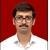true

Take Class 12 Tuition from the Best Tutors

•Affordable fees
•1-1 or Group class
•Flexible Timings
•Verified Tutors

Search in

# Balancing Redox ReactionDr. Nishant Khandelwal
26/05/2021 00

Redox reaction is an Oxidation-Reduction Reaction. Balancing a redox reaction in an essential medium requires an additional step when we finish of which involves the addition of an equal number of hydroxyl ions to both sides of the equation, followed by combining the H+ and OH- to make H2O and cancelling out the water molecule that is present on both sides.

Balancing involves the following steps:

Step 1: Write the unbalanced ionic equation. Fe(s) + CrO42- (aq) → Fe2O3 (s) + Cr2O3 (s)

Step 2: Write separate half-reactions for the oxidation and the reduction processes.

Oxidation : Fe (s) → Fe2O3  (aq)          Fe is in +3 oxidation state here Fe2O3  (aq)

Fe0 (s) → Fe+3 (aq)

The oxidation state of iron changes from 0 to +3; therefore, Iron is Oxidised.

Reduction  CrO42- (aq) → Cr2O3 (s); Cr+6 (aq)  → Cr+3 (s)

The oxidation state of Chromium changes from +6 to +3; therefore, Chromium is reduced.

Step 3: Balance the atoms in the half-reactions other than the hydrogen and oxygen.

2 Fe (s)  → Fe2O3 (aq); 2 CrO42- (aq) → Cr2O3 (s)

Step 4: Balance oxygen atoms by adding water molecules to the respective side of the equation.

2 Fe (s) + 3H2O(l) → Fe2O3 (aq); 2 CrO42- (aq) → Cr2O3 (s) + 5H2O(l)

Step 5: Balance Hydrogen atoms by adding H+ ions to the respective side of the equation.

2 Fe (s) + 3H2O(l) → Fe2O3 (aq) + 6 H+(aq); 10 H+(aq) + 2 CrO42- (aq) → Cr2O3 (s) + 5H2O(l)

Step 6: Balance the charges by adding electrons to each half-reaction and then equalise the electrons by multiplying with a coefficient.

2 Fe (s) + 3H2O (l)→ Fe2O3 (aq) + 6 H+(aq) + 6e- ( Fe0 --> Fe3+ + 3e- ) x 2

6e- + 10 H+ (aq) + 2 CrO42- (aq) → Cr2O3 (s) + 5H2O(l) (  Cr+6 (aq) + 3e- → Cr+3 (s) ) x 2

Step 7: a) Add the two half-reactions together. b) The electrons must cancel.

2 Fe (s) + 3H2O(l) → Fe2O3 (aq) + 6 H+ (aq)+ 6e-                  Oxidation

6e-   + 10 H+ (aq)+ 2 CrO42- (aq) → Cr2O3 (s) + 5H2O(l) Reduction

------------------------------------------------------------------------------------------------

2 Fe (s) + 3H2O(l) + 10 H+ (aq)+ 2 CrO42- (aq) → Fe2O3 (aq) + 6 H+ (aq)+ Cr2O3 (s) + 5H2O(l)

c) Balance any remaining substances by inspection, cancel out H2O or H+ that appear on both sides if necessary.

2 Fe (s) +  4 H+ (aq)+ 2 CrO42- (aq) → Fe2O3 (aq) +Cr2O3 (s) + 2H2O(l)

Step 8: Adding OH- hydroxide ions on both sides equivalent to H+ ions.

4 OH-(aq)  + 2 Fe (s) +  4 H+ (aq)+ 2 CrO42- (aq) → Fe2O3 (aq) +Cr2O3 (s) + 2H2O(l) + 4 OH-(aq)

Step 9: Combining OH- ions and H+ ions to form water.

2 Fe (s) +  4 H2 O(l) + 2 CrO42- (aq) → Fe2O3 (aq) +Cr2O3 (s) + 2H2O(l) + 4 OH-(aq)

Step 10:  Cancelling out water molecule, if any.

2 Fe (s) +  4 H2 O(l) + 2 CrO42- (aq) → Fe2O3 (aq) +Cr2O3 (s) + 2H2O(l) + 4 OH-(aq) gives 2 Fe (s) +  2 H2 O(l) + 2 CrO42- (aq) → Fe2O3 (aq) + Cr2O3 (s) + 4 OH-(aq)

Now we have our Balanced Redox reaction in basic medium:

2 Fe (s) +  2 H2 O(l) + 2 CrO42- (aq) → Fe2O3 (aq) + Cr2O3 (s) + 4 OH-(aq)

The equation is finally balanced by atoms and by charge, The presence of hydroxide ions means that the reaction takes place in basic solution.

0 Dislike
Follow 2Please Enter a comment

## Other Lessons for You

CONCEPT OF HYBRIDIZATION
Hybridisation is a hypothetical concept which explains about the formation and structure of molecules. Defination - the intermixing of atomic orbitals to produce symmetrical low energy containg hybrid...Isotopes-1
1) What is an Isotope? Isotopes are atoms of the same element that have different numbers of neutrons but the same number of protons and electrons. 2) What determines the atomic number of an element? A...Conditions for the transfer of heat energy
Whenever there is a temperature difference, there is a transfer of heat energy occurring between two material bodies. Conditions for the transfer of heat energy to occur: Condition 1: Material bodies...
C

Inert Pair Effect
On moving down in a group in p-block the higher valency becomes less stable and lower valencies become more stable. For example: Stability : C+4 > Si+4 > Ge+4 > Sn+4 > Pb+4 ...Writing Chemical Formula
WRITING CHEMICAL FORMULA- *The Chemical Formula is a representation of a substance by means of the symbol. THE RULES FOR WRITING CHEMICAL FORMULA ARE- *The...### Looking for Class 12 Tuition ?

Learn from Best Tutors on UrbanPro.

Are you a Tutor or Training Institute?

Join UrbanPro Today to find students near you

X

### Looking for Class 12 Tuition Classes?

The best tutors for Class 12 Tuition Classes are on UrbanPro

• Select the best Tutor
• Book & Attend a Free Demo
• Pay and start Learning### Take Class 12 Tuition with the Best Tutors

The best Tutors for Class 12 Tuition Classes are on UrbanProUrbanPro.com is India's largest network of most trusted tutors and institutes. Over 55 lakh students rely on UrbanPro.com, to fulfill their learning requirements across 1,000+ categories. Using UrbanPro.com, parents, and students can compare multiple Tutors and Institutes and choose the one that best suits their requirements. More than 7.5 lakh verified Tutors and Institutes are helping millions of students every day and growing their tutoring business on UrbanPro.com. Whether you are looking for a tutor to learn mathematics, a German language trainer to brush up your German language skills or an institute to upgrade your IT skills, we have got the best selection of Tutors and Training Institutes for you. Read more